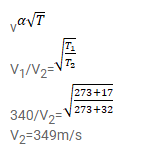# The speed of sound as measured by a studentQuestion:

The speed of sound as measured by a student in the laboratory on a winter day is $340 \mathrm{~m} \mathrm{~s}$ when the room temperature is ${\mathrm{C} 17^{\circ}}^{\circ}$. What speed will be measured by another student repeating the experiment on a day when the room temperature is $32^{\circ} \mathrm{C}$ ?

Solution: